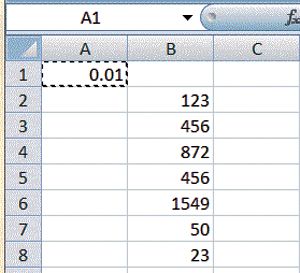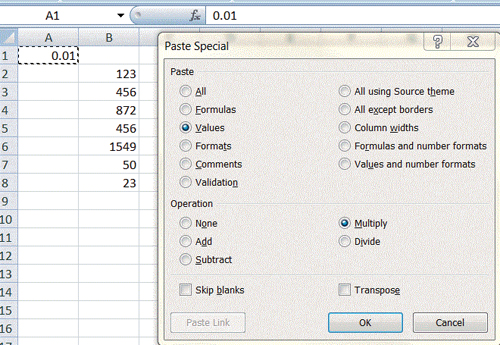Technology

# A Global Shift (of Decimals)

How to move the decimal point in an array of Excel data.

Q: I have a column in a spreadsheet that mistakenly has whole numbers instead of percentages — for instance, 123 instead of 1.23. How can I shift the decimal point two positions to the left?

A: Follow these steps to turn whole numbers into decimals or percentages:

Go to any blank cell in the spreadsheet and enter 0.01.

Copy that cell, which puts 0.01 on the clipboard (Figure 1).

Select all the cells in your spreadsheet that you want to convert to percentages. Right-click and choose Paste Special. When the dialog box pops up, choose Values and Multiply and OK (Figure 2).

This will multiply all of the selected cells by 0.01, and 123 will become 1.23.

If you would like to submit a question to Bill “MrExcel” Jelen, go to CFO’s Spreadsheet Community Center at www.cfo.com/spreadsheets.

Figure 1Figure 2